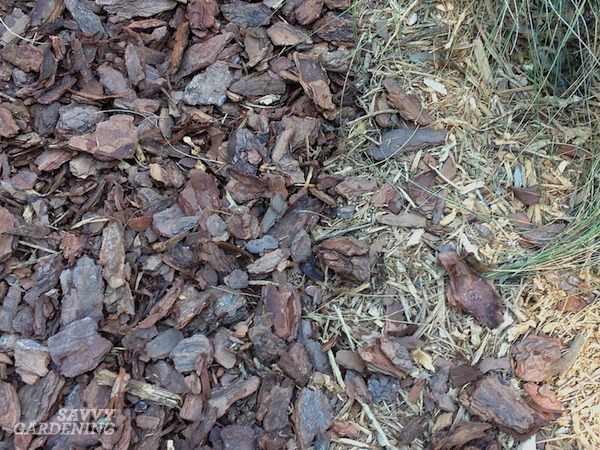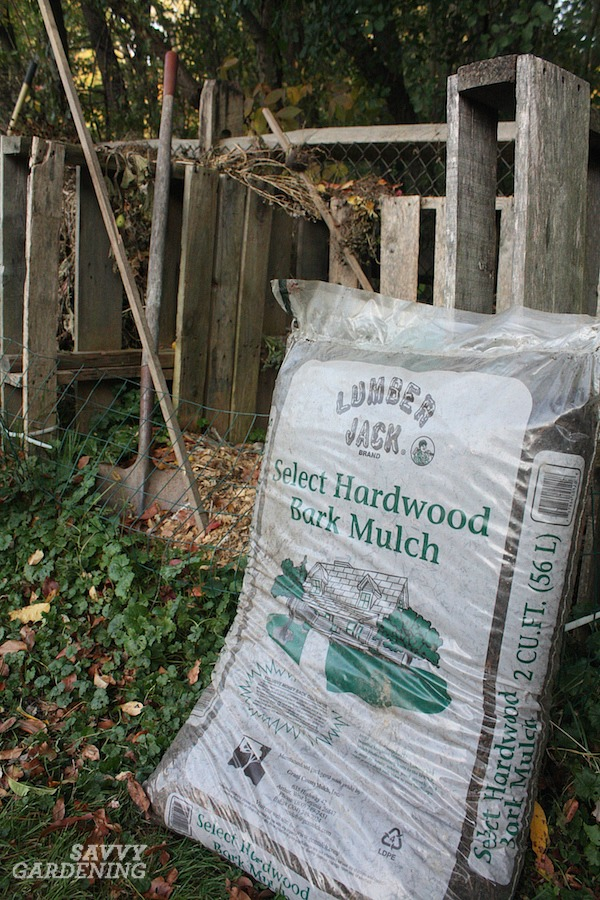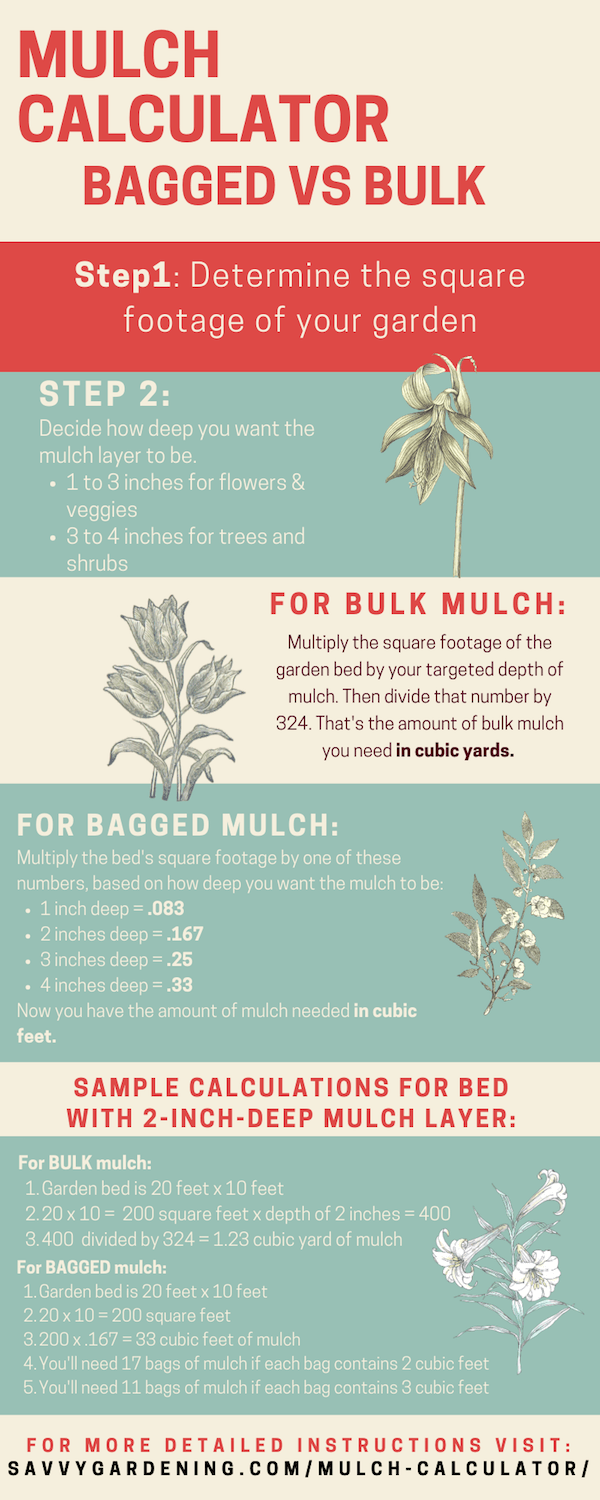# Mulch calculator: How to determine the amount of mulch you need

This post may contain affiliate links. If you click on an affiliate link and make a purchase, we receive a small commission at no extra cost to you. Find our full disclosure here.

Mulching is an essential spring chore for every gardener. Whether you’re mulching the vegetable garden to cut down on watering, dressing up your flower beds, or hoping to keep weeds at bay in shrub plantings, it’s important to know how much mulch you need for the job. Getting stuck with too little mulch – or worse, too much! – has caused plenty of gardeners to scratch their head in confusion. This article introduces mulch calculator formulas you can use to purchase the perfect amount of mulch for your garden each and every time.

## Step by step mulch calculator

Follow this simple plan to calculate how much mulch you’ll need for your garden and landscape. The only tools you’ll need are a measuring tape or a measuring wheel and the calculator on your cell phone.

## Step 1: Decide on the type of mulch to use

There are many different types of landscape mulch, from shredded bark and pine chips to compost and pine straw. It’s a smart move to base the type of mulch you use on which plants are growing in the area and what’s available to you on the local level. If you’re unsure of which type of landscape mulch to use, you can read all about different kinds of mulches and where to use them in this article.

Once you’ve decided which type of mulch you’ll be using, the following mulch calculator formulas will help you calculate how much you’ll need for any type of mulch.There are many different types of mulch for planting beds, including pine bark nuggets and arborist wood chips.

## Step 2: Determine the square footage of the garden area.

After you’ve settled on which type of mulch you’re going to use, it’s time to determine how big the area is that you want to mulch.

• To calculate the square footage of a square or rectangular garden area, multiply its height (in feet) times its width (in feet).

W x H = square footage of a rectangular area

• To calculate the square footage of a circular area, measure the distance from the center of the bed to the outer edge (this is the radius, R). Then multiply that number by itself. Then multiply the total by 3.14 (π)

R x R x 3.14 = square footage of a circular area

If your garden bed is an odd shape, such as a kidney bean or a wide oval, that’s okay. Just do your best to divide it into a series of imaginary rectangles and circles put together to make that shape. Then calculate the square footage of each of those pieces and add them together.

***If you have multiple areas you’d like to mulch in different parts of your yard, determine the square footage of each separate area and then add those numbers together to get the total square footage you want to cover.For oddly shaped garden beds, mentally divide them into standard shapes to calculate their square footage.

## Step 3: Decide how deep you want the mulch layer to be

Now that you know the total square footage of your bed, determine how deep you’d like your mulch layer to be. For tree and shrub beds, I suggest 3 to 4 inches of mulch (when it comes to mulching these plants, more is never better since thick layers and restrict oxygen flow to the root zone). For vegetable and flower gardens, 1 to 3 inches is best.

To make the process easier, I’m now going to give you two different options, based on whether you plan to purchase your mulch in bags or buy it in bulk.

## Bagged mulch vs a truckload of mulch

Here in the US, bagged mulch is sold in bags that are measured in cubic feet, but mulch sold by the truckload is measured in cubic yards. So, how do you know whether it’s more cost effective to purchase bags of mulch or order a bulk delivery that arrives in the bed of a truck? I’ll give you a mulch calculator formula for both bagged mulch and bulk mulch to make the process easy. Once you’ve determined how much mulch you need using both bags and bulk, you can then price them both out to see what’s less expensive.Bulk mulch amounts are calculated by following the steps below.

## Step 4: BULK mulch calculator formula

Use this mulch calculator formula to figure out how much mulch you need when buying in bulk.

Now that you know your square footage and your desired mulch thickness, it’s time to crunch the numbers (don’t worry, it isn’t hard).

To determine how much mulch you need if you’re buying it by the truckload:

1. Multiply the square footage of the bed by the number of inches of mulch you want on top of it
2. Divide that number by 324

Now you have the amount of mulch needed in cubic yards.

For example, if your bed is 50 feet long by 10 feet wide, the square footage is 500 square feet. If you want the mulch to be 2 inches deep, you’ll need 3 cubic yards of mulch.

50 x 10 x 2 = 1000. Then 1000 divided by 324 = 3.08 cubic yards.Calculating the amount of mulch bags you need to purchase is easy when you follow the steps in the next section.

## Step 5: BAGGED mulch calculator formula

Use this mulch calculator formula to figure out how much mulch you need when buying by the bag.

To determine how much mulch you need if you’re buying it in bags measured in cubic feet, follow this step:

1. Multiply your square footage by one of these numbers, based on how deep you want the mulch to be:
1 inch deep = .083
2 inches deep = .167
3 inches deep = .25
4 inches deep = .33
5 inches deep = .417

Now you have the amount of mulch needed in cubic feet.

For the sample bed that was 50 feet by 10 feet (500 square feet), if you want the mulch to be 2 inches deep, you’ll need 83.5 cubic feet of mulch.

50 x 10 = 500. Then 500 x .167 = 83.5 cubic feet of mulch.

If the bag of mulch holds 2 cubic feet, you’ll need to buy 42 bags (83.5 divided by 2)
If the bag of mulch holds 3 cubic feet, you’ll need to buy 28 bags (83.5 divided by 3)Some bags of mulch inform you of the size of area covered by the bag, but most do not.

## What’s more cost effective, mulch by the bag or in bulk?

Now that you’ve used the mulch calculator, it’s easy to determine whether it would be more economical to buy your mulch in bags or by the truckload. Using the above example, I think it would be a bit ridiculous to purchase 42 bags of mulch, simply for the amount of plastic bags headed to the landfill. But, it’s also more expensive, too. Here’s why:

• A 2-cubic-foot bag of shredded hardwood mulch costs an average of \$3.99 where I live. At that price, 42 bags would cost \$167.58.
• A cubic yard of bulk shredded hardwood mulch from our local landscape supply yard costs \$32.00. For the 3 cubic yards I’d need for my 50 ft x 10 ft bed, the total price would be \$96.00.

You don’t need to be a math whiz to see that, in this example, buying in bulk is substantially cheaper than buying bags. In some other situations, buying bags may be cheaper. The only way to find out is to compare the two options.

For your own garden, do the calculations both using bags and using bulk and price them out before you spend a single cent on mulch this spring. When used right, this mulch calculator system keeps you from blowing your entire gardening budget on mulch…. and leaves you more \$\$\$ to spend on PLANTS! And everyone needs more plants, right?!?

Pin this infographic and you’ll always have this information on your favorite Pinterest board!